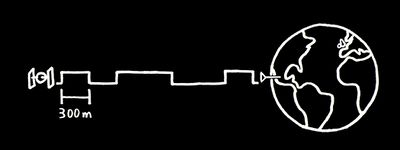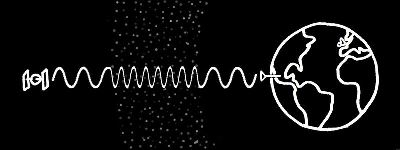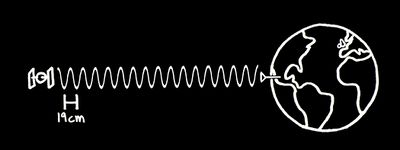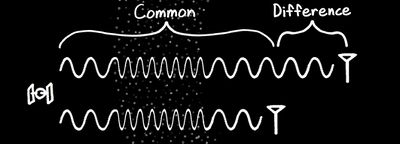## Comparison : Standard GPS/GNSS Receiver vs RTK GPS/GNSS System

 Standard GPS/GNSS Receiver RTK GPS/GNSS System Number of Receivers One : Roving Receiver Two : Roving Receiver and Reference Receiver Position Type Absolute (Latitude, Longitude, Altitude) Absolute (Latitude, Longitude, Altitude)andRelative (vector between reference and rover) Time To First Fix 30 - 60 s 40 - 70 s Horizontal Position Accuracy 3 - 5 m 1 - 5 cm Vertical Position Accuracy 12 - 15 m 8 - 15 cm

## How does GPS/GNSS positioning work?

A GPS/GNSS receiver determines its position by measuring its distance to four or more GPS, GLONASS, BeiDou or Galileo satellites.

In order to determine its distance to the satellites, the receiver measures the phase of unique 'codes' continuously transmitted by the satellites. By comparing the relative phase offsets of the received codes, the receiver can determine the relative distance to each satellite, i.e. the distance to each satellite plus a common offset (the time of the measurement is unknown until solved for, leading to an unknown common offset to all the distances).

Once the relative distances to the satellites are known, the three dimensional coordinates of the receiver and the time of the measurement can then be solved for via iterative algorithms.Fig 1. GPS/GNSS receiver measuring the distances to four satellites; the minimum for a 3D position fix.

## Why is a typical GPS/GNSS receiver only accurate to a few meters?

The phase of the code must be measured very precisely in order to correctly measure the distance to the satellite. In practice, there is a limit to the precision with which the code phase can be measured. Each bit of the codes transmitted by the satellites is quite long - about 300 meters in length. The 300 meter of the code bits combined with the imprecise code phase measurement leads to an upper bound on the accuracy with which the distance to the satellite can be measured - generally a few meters.Fig 2. To determine the distance to a satellite, a receiver measures the phase of the code continuously transmitted by that satellite. Each bit of the code is about 300 meters long.

Another important source of error is ionospheric delay. The ionosphere, a layer of charged particles surrounding the earth, slows the GPS signals as they pass through it. This delay is hard to estimate and varies by time and location, leading to a few meters of error in the measurement of the distance to the satellite.Fig 3. The ionosphere slows GPS signals, adding a few meters of error in the distance measurement.

## How does RTK GPS/GNSS positioning work?

RTK GPS/GNSS systems are able to achieve much higher positioning precision by mitigating the sources of error described above.

### Carrier Measurement

First, in addition to measuring the code phase, an RTK receiver measures the phase of the carrier wave that the code is modulated upon. The carrier has a wavelength of about 19 centimeters. This makes it possible to measure to a much greater degree of accuracy than the 300 meter code.

However, there is a catch - there are an unknown number of whole carrier wavelengths between the satellite and receiver. Clever algorithms are required to resolve this "integer ambiguity" by checking that the code and carrier phase measurements lead to a consistent position solution as the satellites move and the geometry of the problem changes.Fig 4. The carrier, at 19 centimeters per wavelength, is much more precise than the code.